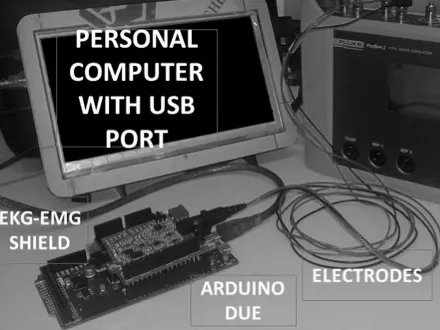Project tutorialHeart Rate Estimation Using Arduino DUE © CERN-OHL

This work the design and implementation of a digital system based on open hardware development board applied to heart rate estimation.

• 250 views
• 0 respects

Components and supplies

The electrocardiogram or ECG signal is the principal record used for anomalies detection of the human heart. The ECG is an electrical signal with low amplitude and is composed for different waves form. ECG signal is acquired from human body surface using amplifier and filter analog circuits. But, some noise components not allows the detection or the waves form. Whereby, a filtering strategies and wave form detection algorithms are required in the ECG treatment. The detection of R wave from ECG signal is the most important reference for heart rate estimation and is the principal object in this paper.

This work the design and implementation of a digital system based on open hardware development board (Arduino) applied to heart rate estimation. Positive results are presented about digital filtering oriented to R wave detection and thresholding based on histogram calculation from ECG signal. Results from test using patient simulator with different heart rate values are shown and its error percentage.

Code

Complete ProgramC/C++
This is the program for heart rate estimation.
#include <SPI.h>
#include <SD.h>
const int chipSelect = 4;
int N=3000;
int n=0;
int muestra;
int x={0};
int x1={0};
int h={0};
int d={0};
int xr;
int M0;
int M1;
int i;
int c;
int L;
int a=0;
int u=0;
int s=0;
int ini=0;
int m=0;
int temp;
int z=0;
int r=0;
int p=0;

void setup()
{
L=32;
Serial.begin(9600);
Serial.print("Initializing SD card...");
// see if the card is present and can be initialized:
if (!SD.begin(chipSelect))
{
Serial.println("Card failed, or not present");
return;
}
Serial.println("card initialized.");

//////////////////

for(n=0;n<N;n++)
{
delay(1);
}

for (n=L;n<N;n++)
{
c=0;
for (i=0;i<L;i++)
{
c=c+x[n-i];
}
x1[n]=c >> 4;
}

x=0;

for (n=1;n<N;n++)
{
c=x1[n]-x1[n-1];
if (c < 0) c=0;
if (c > 1024) c=0;
x[n]=c;
}

/////////////

for(n=0;n<N;n++)
{
File dataFile = SD.open("ELECTRO.txt", FILE_WRITE);
String dataString = "";
muestra = x[n];
h[muestra]=h[muestra]+1;
dataString += String(muestra);
dataFile.println(dataString);
dataFile.close();
}

for(n=0;n<1100;n++)
{
File dataFile = SD.open("HISTO.txt", FILE_WRITE);
String dataString = "";
muestra = h[n];
dataString += String(muestra);
dataFile.println(dataString);
dataFile.close();
}

d=0;
for (n=1;n<1100;n++)

{
File dataFile = SD.open("DERIV.txt", FILE_WRITE);
String dataString = "";
d[n]=h[n]-h[n-1];
dataString = String(d[n]);
dataFile.println(dataString);
dataFile.close();
}

c=0;
p=0;
for (n=1;n<1100;n++)
{
if (h[n]>c)
{
c= h[n];
p=n;
}
}

for (n=p;n<1100;n++)
{
if (h[n]==0)
{
c= n;
break;
}
}

Serial.println("Valor Umbral");
Serial.println(c);

for (n=0;n<N;n++)
{
File dataFile = SD.open("UMBRAL.txt", FILE_WRITE);
String dataString = "";
if (x[n]>c)
{
x[n]=1;
}
else
{
x[n]=0;
}
dataString = String(x[n]);
dataFile.println(dataString);
dataFile.close();
}

for (n=0;n<N;n++)
{

if (x[n]==1)
{
ini=n;
break;
}

}

Serial.println(ini);

for (m=ini;m<N;m++)
{
if (x[m]==0)
{
temp=temp+1;
z=0;
}
else
{
z=z+1;
if (z<=1)
{
r=r+1;
xr[r]=temp;
temp=0;
z=z+1;
}
else
{
z=z+1;
temp=temp+1;
}
}
}

Serial.println(r);

for (n=0;n<10;n++)
{

File dataFile = SD.open("FREC.txt", FILE_WRITE);
String dataString = "";
dataString = String(xr[n]);
dataFile.println(dataString);
dataFile.close();
}

}

void loop() {

}

Custom parts and enclosures

This is the ECG shield from OLIMEX used as analog amplifierThis was used as ECG signal generator. This is profesional tool for testing hear estimation systemsSchematics

paper_DaFCvWe7DF.docx

Author

• Designer by Javier Gonzalez
• Deigner by Alejandra Urbano

Published on

August 12, 2019
See similar projects
you might like

Heart Rate Monitor (Wearable and Wireless Using ECG)

Project tutorial by Dmitry Dziuba

• 7,337 views
• 29 respects

Heart Rate Monitor Using IoT

Project tutorial by Team Technopaths

• 18,648 views
• 31 respects

Detecting Heart Rate with a Photoresistor

Project tutorial by 3 developers

• 12,918 views
• 37 respects

Measure Heart Rate and SpO2 with MAX30102

Project tutorial by SurtrTech

• 33,328 views
• 134 respects

Heart Rate Monitoring System

Project tutorial by Team HRMS

• 20,453 views